NCERT Solutions: How Many Squares

# NCERT Solutions: How Many Squares - Notes | Study Mathematics for Class 5: NCERT - Class 5

 1 Crore+ students have signed up on EduRev. Have you?

NCERT Solution, How many Squares, class 5, Mathematis

1. Ziri tried to make some other tiles. She started with a square of 2 cm side and made shapes like these. Look at these carefully and find out:

Q. Which of these shapes will tile a floor (without any gaps)? Discuss. What is the area of these shapes?

Q. Make designs in your copy by tiling those shapes.

Q. Now you create your own new tiles out of a square. Can you do the same with a triangle? Try doing it.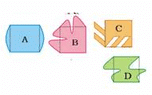Ans. The shapes C and D will tile a floor (without any gaps)

The area of each of these shapes (i.e. tiles) is

2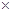2 square cm = 4 square cm.

2. Make a pattern using your tile. Trace the shape to repeat it on a page, but remember there must be no gaps between them. Ziri made a pattern using her yellow tiles. (You know the area of her tile.)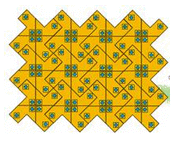Q. How many tiles has she used?

Q. What is the area of the floor pattern Ziri has made here?

Ans. She has used 12 tiles.

The area of the floor pattern Ziri has made here is (123) square cm= 36 square cm.

3. Did you get all the 12 shapes using 5 squares?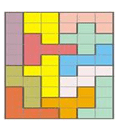Draw all the 12 shapes on a sheet of cardboard and cut them.

Ans. Yes, I get all the 12 shapes using 5 squares as shown above.

All the 12 shapes may be drawn on a sheet of cardboard and also cut them as desired.

4. Ziri went to a shop and was surprised to see the different designs of tiles on the floor. Aren’t these beautiful! Can you find the tile which is repeated to make each of these floor patterns? Circle a tile in each pattern.

Ans. A tile in each pattern is shown as encircle.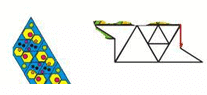5. After looking at the patterns Ziri wanted to make her own yellow tile. You too make a tile this way.

Step 1: Take a piece of cardboard or thick paper. Draw a square of side 3 cm on it.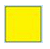Step 2: Draw a triangle on any one of the sides of this square.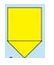Step 3: Draw another triangle of the same size on another side of the square. But this time draw it inside the square.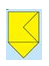Step 4: Cut this shape from the cardboard. Your tile is ready! What is it’s area?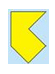Ans. It’s area is 33 square cm i.e. 9 square cm.

6. Here is a rectangle of area 20 square cm.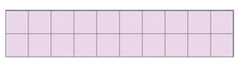a) Draw one straight line in this rectangle to divide it into two equal triangles. What is the area of each of the triangles?

b) Draw one straight line in this rectangle to divide it into two equal rectangles. What is the area of each of the smaller rectangles?

c) Draw two straight lines in this rectangle to divide it into one rectangle and two equal triangles.

d) What is the area of the rectangle?

e)  What is the area of each of the triangles?

Ans. (a) One straight line is drawn in the given rectangle to divide it in two equal triangle as: shown below.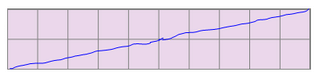Area of each of the triangles is half of the given rectangle. Area of this rectangle is 20 square cm. So, the area of each of the triangles is 10 squares cm.

(b) One straight line is drawn in the given rectangle to divide it into two equal rectangles as shown below: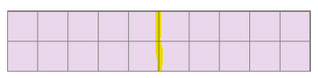Area of each of the smaller rectangles is half of the given rectangle. Area of the given rectangle is 20 square cm. So, the area of each of two rectangles is 10 square cm.

(c) Two straight lines are drawn in the given rectangle to divide it into one rectangle and two equal triangles as shown below: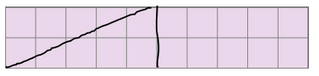(d) Area of the new rectangle is half of the given rectangle. Area of the given rectangle is 20 square cm. So, the area of the smaller rectangle is 10 square cm.

(e) Area of the each of the triangles is half of the rectangle of area 10 square cm. So, the area of each of the triangles is 5 square cm.

The document NCERT Solutions: How Many Squares - Notes | Study Mathematics for Class 5: NCERT - Class 5 is a part of the Class 5 Course Mathematics for Class 5: NCERT.
All you need of Class 5 at this link: Class 5

## Mathematics for Class 5: NCERT

36 videos|73 docs|45 tests
 Use Code STAYHOME200 and get INR 200 additional OFF

## Mathematics for Class 5: NCERT

36 videos|73 docs|45 tests

Track your progress, build streaks, highlight & save important lessons and more!

,

,

,

,

,

,

,

,

,

,

,

,

,

,

,

,

,

,

,

,

,

;